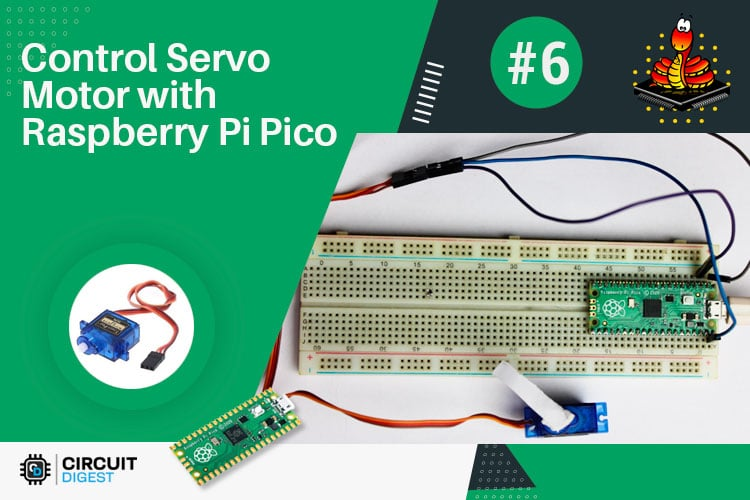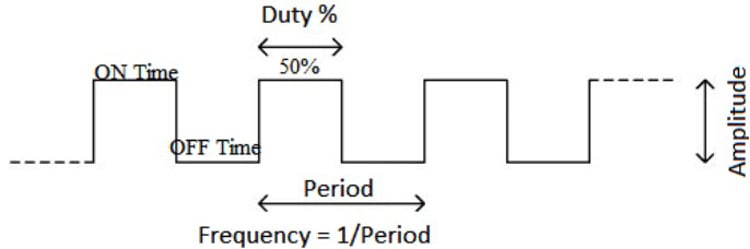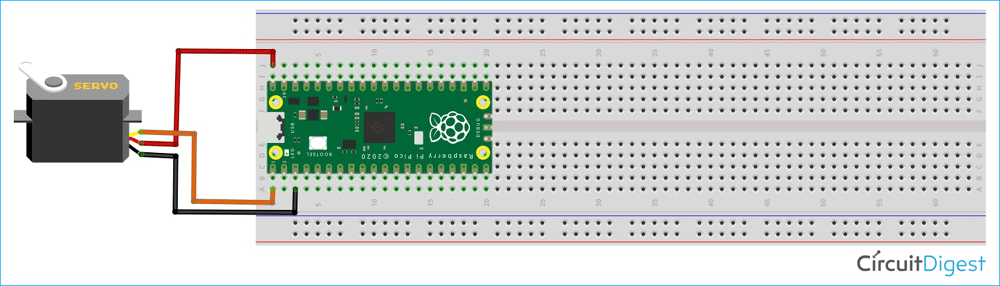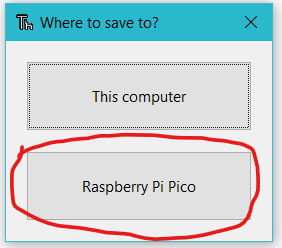# Control a Servo Motor with Raspberry Pi Pico Using PWM in MicroPython

Published  September 24, 2021   0
J
AuthorWith the hope that you are enjoying our Raspberry Pi Pico tutorial series, we are here with another interesting tutorial. In this tutorial we are going to control a servo motor with Raspberry Pi Pico using the Pulse Width Modulation in MicroPython. So far, we have learnt how to interface an OLED and LCD. Along with that, we also got to learn how to implement the I2C, ADC communication with Raspberry Pi Pico. Let us now understand how a pulse width modulation works.

### Pulse Width Modulation

Pulse-width modulation (PWM) is a technique for changing the amplitude of digital signals to control devices and applications that require power or electricity. It effectively regulates the amount of power delivered to a device from the standpoint of the voltage component by rapidly cycling the on-and-off phases of a digital signal and changing the breadth of the "on" phase or duty cycle. To the device, this would appear as a constant power input with an average voltage value determined by the proportion of on time. The duty cycle is represented as a percentage of being totally (100%) on.The following image represents a PWM signal with 50% duty cycle. We can control the “on time” of PWM signal by varying the duty cycle from 0% to 100%. We are going to control a servo motor by varying the duty cycle of a particular PWM signal. One Period is the complete ON and OFF time of a PWM signal as shown in the above figure. The frequency of a PWM signal determines how fast a PWM completes one period. The formulae to calculate the Frequency is given below

```Frequency = 1/Time Period
Time Period = On time + Off time```

Normally, the PWM signals generated by microcontroller will be around 500 Hz, such high frequencies will be used in high-speed switching devices like inverters or converters. But not all applications require high frequency. For example, to control a servo motor, we need to produce PWM signals with 50Hz frequency, so the frequency of a PWM signal is also controllable by program for all microcontrollers. For more information, you can check our What is PWM: Pulse Width Modulation article.

### Circuit Diagram of the Servo Motor with the Raspberry Pi PicoThe connection of a servo motor is really simple. We know that a servo motor has three wires. The black or brown wire is for ground, the red wire is used as VCC and the yellow or orange wire is used to provide the pulse signal. In the circuit diagram, you can see that we have connected the VCC of the servo with the VBUS pin of the Pico board. The Signal pin of the servo motor has been connected with the GPIO0 of the Pico board and the Ground pin of the servo has been connected to the ground pin of the Pico board.

### Raspberry Pi Pico Servo Motor Control Code in MicroPython

You need to clone the Raspberry Pi Pico Tutorial GitHub repository.  Open the “T6_How to Control a Servo Motor using Pico” folder. Inside this folder, you can find the “code” folder. Open the “main.py” python file in the Thonny editor. Now, let’s discuss about the main.py file.

```from machine import Pin, PWM
from time import sleep```

At first, we need to import the Pin() and PWM() classes from the machine.py library as mentioned above. In our previous tutorials, we have used machine library so many times and I hope you are now familiar with the machine library. The Pin() is used to declare the signal pin of the servo motor.

```pwm = PWM(Pin(0))
pwm.freq(50)```

As I have connected the signal pin of the servo motor to the GPIO0 of the Pico board, I have declared the Pin(0) and setup this pin as PWM by passing it into the PWM() function. The pwm variable is used as an object here. Then I have assigned the frequency as 50Hz by using the pwm.freq(50).

```#Function to set an angle
#The position is expected as a parameter
def setServoCycle (position):
pwm.duty_u16(position)
sleep(0.01)```

The setServoCycle(position) function can be used to set the position of the servo by passing the position parameter from 1000 to 9000. The values for duty_u16 are in microseconds instead of degrees. The servo values 1000-9000 represent 0-180 degrees.

```while True:
for pos in range(1000,9000,50):
setServoCycle(pos)
for pos in range(9000,1000,-50):
setServoCycle(pos)```

In the above while loop, I am using two for loops. First one is used to set the servo position from 0 to 180 that is 1000 to 9000 and the second for loop is used to set the servo position from 180 to 0 that is 9000 to 1000. Now, in the Thonny IDE, open the “main.py” file. To begin, save the “main.py” file on the Pico board by pressing the “ctrl+shift+s” keys on your keyboard. Before saving the files, make sure your Pico board is connected to your laptop. When you save the code, a popup window will appear, as shown in the image below, you must first select the Raspberry Pi Pico, then name the file “main.py” and save it. This procedure enables you to run the program when the Pico is turned ON.When you upload and run the code on pico board, you will see that the servo is rotating from 0 to 180 degree and 180 to 0 degree continuously. You can refer to the following video below for more details.

Code

from time import sleep
from machine import Pin
from machine import PWM
pwm = PWM(Pin(0))
pwm.freq(50)
#Function to set an angle
#The position is expected as a parameter
def setServoCycle (position):
pwm.duty_u16(position)
sleep(0.01)
while True:
for pos in range(1000,9000,50):
setServoCycle(pos)
for pos in range(9000,1000,-50):
setServoCycle(pos)

Video

Tags